Place Value Table

Chapter 8 Class 6 Decimals
Serial order wise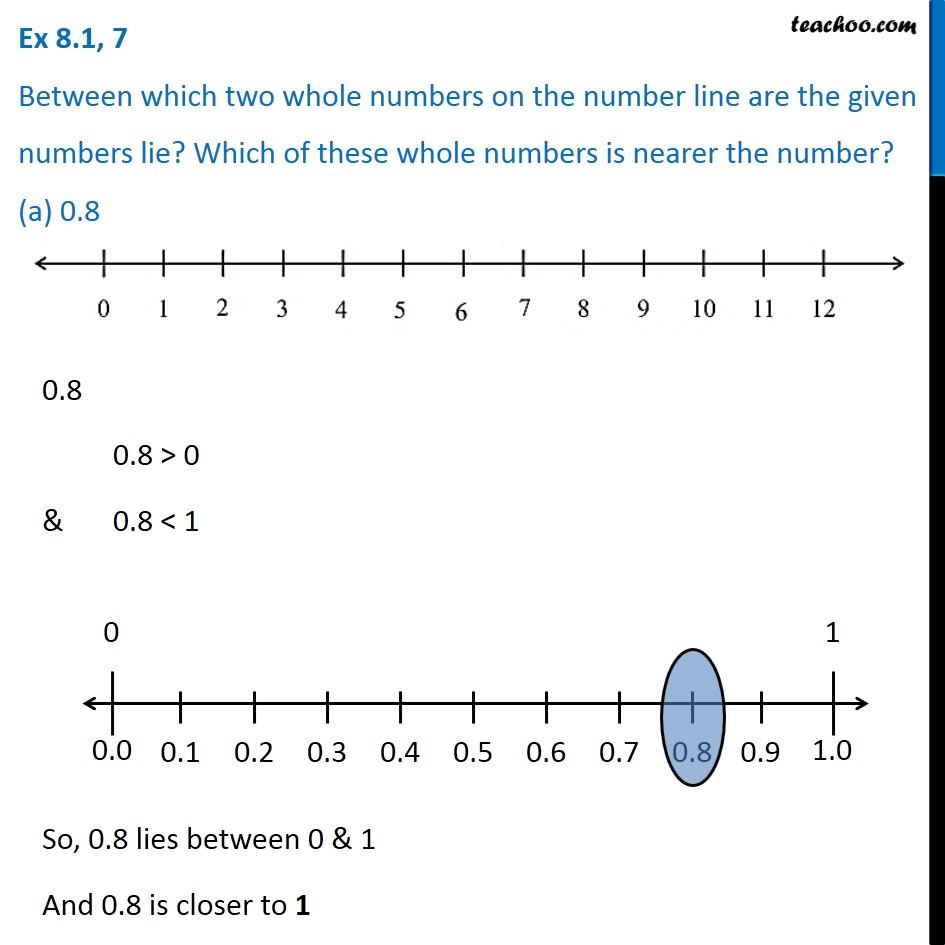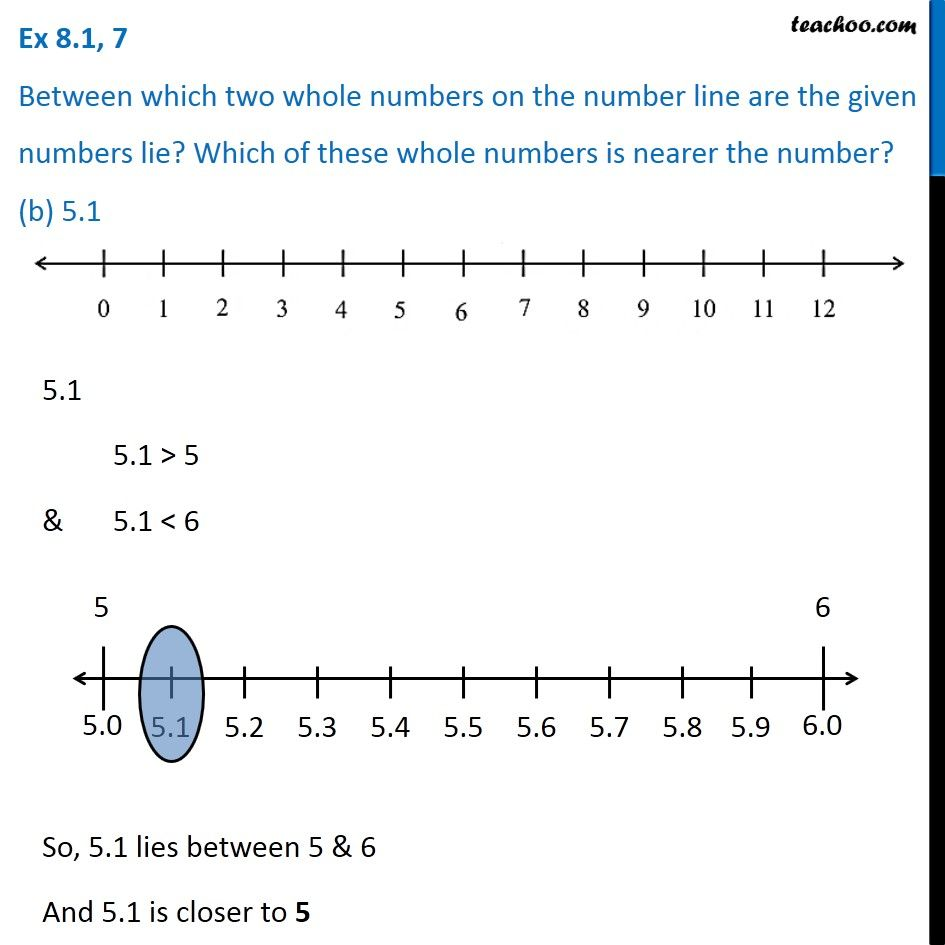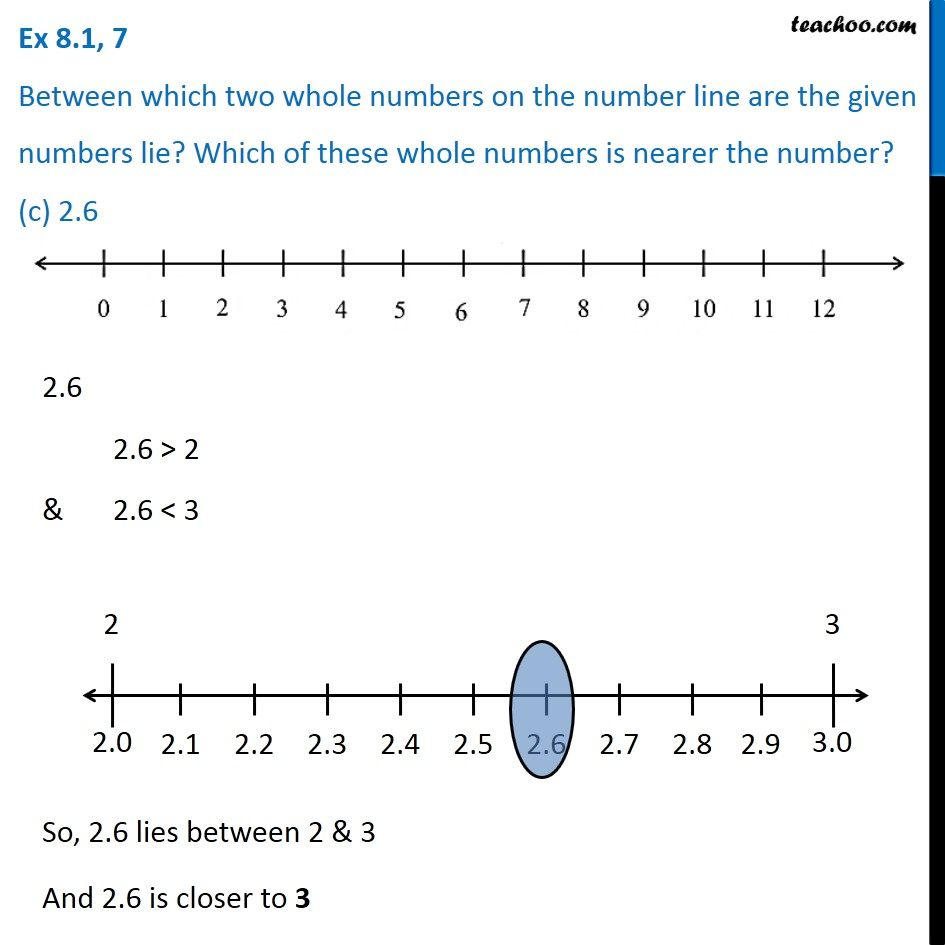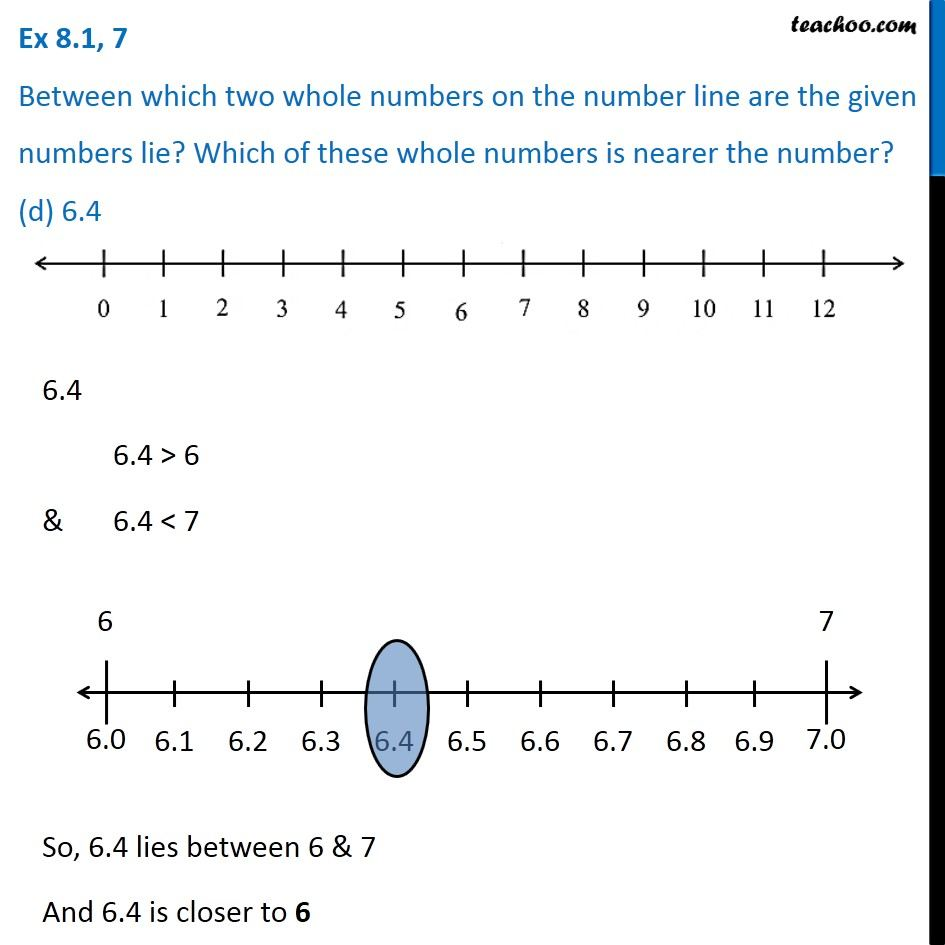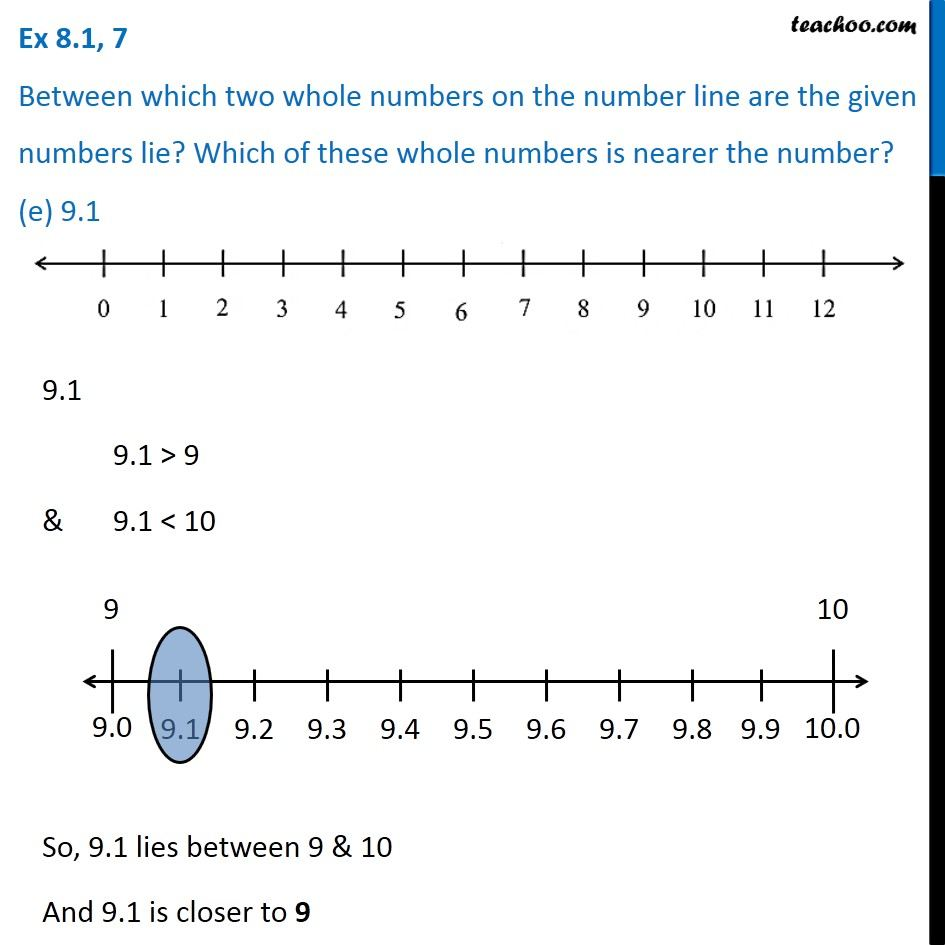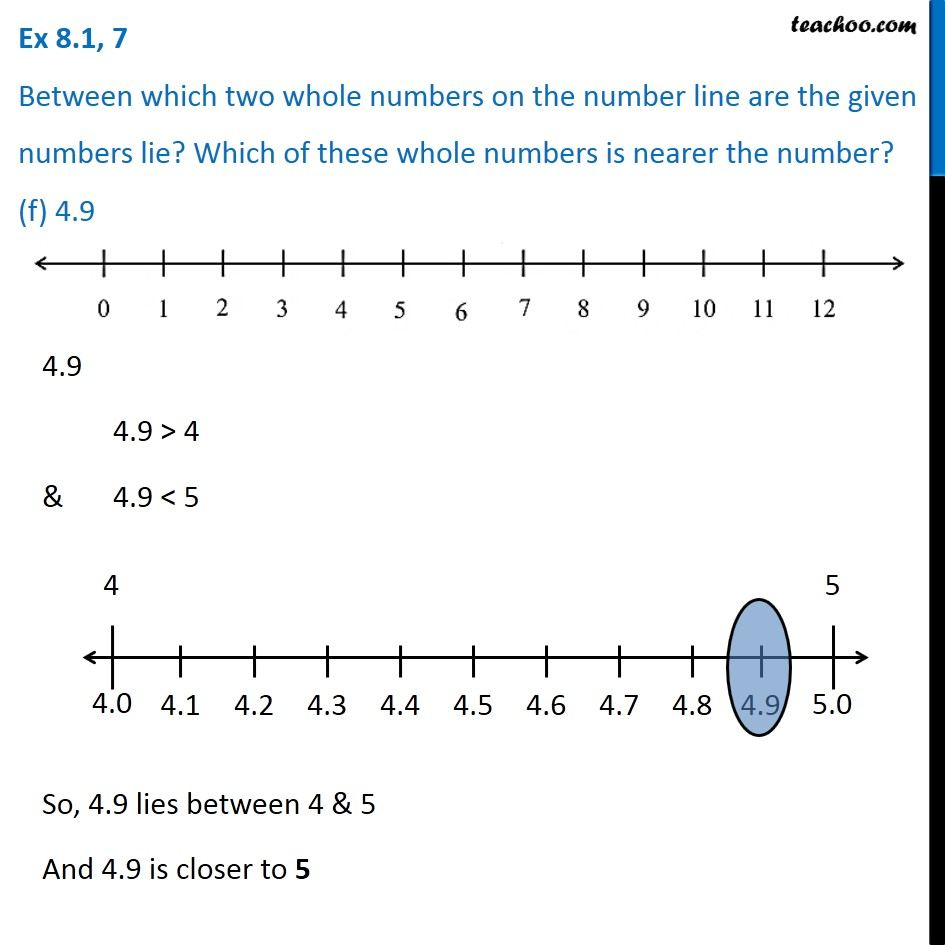Learn in your speed, with individual attention - Teachoo Maths 1-on-1 Class

### Transcript

Question 7 Between which two whole numbers on the number line are the given numbers lie? Which of these whole numbers is nearer the number? (a) 0.8 0.8 0.8 > 0 & 0.8 < 1 So, 0.8 lies between 0 & 1 And 0.8 is closer to 1 Question 7 Between which two whole numbers on the number line are the given numbers lie? Which of these whole numbers is nearer the number? (b) 5.1 5.1 5.1 > 5 & 5.1 < 6 So, 5.1 lies between 5 & 6 And 5.1 is closer to 5 Question 7 Between which two whole numbers on the number line are the given numbers lie? Which of these whole numbers is nearer the number? (c) 2.6 2.6 2.6 > 2 & 2.6 < 3 So, 2.6 lies between 2 & 3 And 2.6 is closer to 3 Question 7 Between which two whole numbers on the number line are the given numbers lie? Which of these whole numbers is nearer the number? (d) 6.4 6.4 6.4 > 6 & 6.4 < 7 So, 6.4 lies between 6 & 7 And 6.4 is closer to 6 Question 7 Between which two whole numbers on the number line are the given numbers lie? Which of these whole numbers is nearer the number? (e) 9.1 9.1 9.1 > 9 & 9.1 < 10 So, 9.1 lies between 9 & 10 And 9.1 is closer to 9 Question 7 Between which two whole numbers on the number line are the given numbers lie? Which of these whole numbers is nearer the number? (f) 4.9 4.9 4.9 > 4 & 4.9 < 5 So, 4.9 lies between 4 & 5 And 4.9 is closer to 5